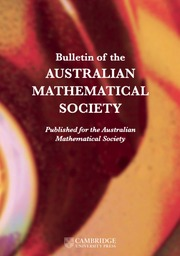Home
Hostname: page-component-768dbb666b-vkhs7 Total loading time: 0.418 Render date: 2023-02-02T12:57:21.748Z Has data issue: true Feature Flags: { "useRatesEcommerce": false } hasContentIssue trueBulletin of the Australian Mathematical Society

# Generalised symmetries of partial differential equations via complex transformations

Published online by Cambridge University Press:  17 April 2009

## Extract

HTML view is not available for this content. However, as you have access to this content, a full PDF is available via the ‘Save PDF’ action button.

We consider two systems of real analytic partial differential equations, related by a holomorphic contact map H. We study how the generalised symmetries of the first equation are mapped into those of the second one, and determine under which conditions on H such a map is invertible. As an application of these results, an example of physical interest is discussed.

Type
Research Article
Information
Bulletin of the Australian Mathematical Society , October 2007 , pp. 243 - 262

## References

Bluman, G.W. and Kumei, S., Symmetries and differential equations, applied mathematical sciences 81 (Springer-Verlag, New York, 1989).CrossRefGoogle Scholar
Bocharov, A. V., Chetverikov, V. N., Duzhin, S. V., Khor'kova, N. G., Krasil'shchik, I. S., Samokhin, A. V., Torkhov, Yu. N., Verbovetsky, A. M. and Vinogradov, A. M., Symmetries and conservation laws for differential equations of mathematical physics, (Krasilśhchik, I. S. and Vinogradov, A. M., Editors) (Amer. Math. Soc., Providence, R.I., 1999).CrossRefGoogle Scholar
Bruhat, F. and Whitney, H., ‘Quelques propriétés fondamentales des ensembles analytiques-réels’, Comment. Math. Helv. 33 (1959), 132160.Google Scholar
Ferraioli, D. Catalano, Manno, G. and Pugliese, F., ‘Contact symmetries of the elliptic Euler-Darboux equation’, Note Mat. 23 (2004), 314.Google Scholar
Dieudonné, J., Foundations of modern analysis (Academic Press, New York and London, 1960).Google Scholar
Grauert, H. and Fritzsche, K., Several complex variables, Graduate Texts in Mathematics 38 (Springer-Verlag, New York, Heidelberg, 1976).CrossRefGoogle Scholar
Krasilśhchik, I.S. and Verbovetsky, A.M., ‘Homological methods in equations of mathematical physics’, (Open Education and Sciences, Opava (Czech Rep.)). math.DG/9808130.Google Scholar
Kulkarni, R.S., ‘On complexifications of differentiable manifolds’, Invent. Math. 44 (1978), 4664.CrossRefGoogle Scholar
Olver, P.J., Application of Lie groups to differential equations (Springer-Verlag, New York, 1993).CrossRefGoogle Scholar
Rogers, C. and Shadwick, W. F., Baecklund transformations and their applications, Mathematics in Science and Engineering, 161 (Academic Press, New York, London, 1982).Google Scholar
Rosenhaus, V., ‘The unique determination of the equation by its invariance group and field-space symmetry’, Algebras Groups Geom. 3 (1986), 148166.Google Scholar
Saunders, D. J., The geometry of jet bundles (Cambridge Univ. Press, Cambridge, 1989).CrossRefGoogle Scholar
Shemarulin, V.E., ‘Higher symmetry algebra structure and local equivalences of Euler-Darboux equations’, (Russian), Dokl. Akad. Nauk. 330 (1993), 2427. Translation in Russian Acad. Sci. Dokl. Math. 47 (1993) 383–388.Google Scholar
Shemarulin, V.E., ‘Higher symmetries and conservation laws of Euler-Darboux equations’, in Geometry in partial differential equations (World Sci. Publishing, River Edge, NJ, 1994), pp. 389422.CrossRefGoogle Scholar
Shemarulin, V.E., ‘Higher symmetry algebra structures and local equivalences of Euler-Darboux equations’, in The interplay between differential geometry and differential equations, Amer. Math. Soc. Transl. Ser. 2 167 (Amer. Math. Soc., Providence, RI, 1995), pp. 217243.Google Scholar
Sparano, G., Vilasi, G. and Vinogradov, A.M., ‘Vacuum Einstein metrics with bidimensional Killing leaves I: local aspects’, Diff. Geom. Appl. 16 (2002), 95120.CrossRefGoogle Scholar
Sparano, G., Vilasi, G. and Vinogradov, A.M., ‘Vacuum Einstein metrics with bidimensional Killing leaves II: global aspects’, Diff. Geom. Appl. 17 (2002), 1535.CrossRefGoogle Scholar
Tognoli, A., ‘Sulla classificazione dei fibrati analitici reali’, Ann. Scuola Norm. Sup. Pisa 21 (1967), 709743.Google Scholar
Tomassini, G., ‘Tracce delle funzioni olomorfe sulle sottovarietà analitiche reali d'una varietà complessa’, Ann. Scuola Norm. Sup. Pisa 20 (1966), 3143.Google Scholar
Vinogradov, A.M., ‘Local symmetries and conservation laws’, Acta Appl. Math. 2 (1981), 2178.CrossRefGoogle Scholar
Vinogradov, A.M., ‘An informal introduction to the geometry of jet spaces’, Rend. Sem. Fac. Sci. Univ. Cagliari 58 (1988), 301333.Google ScholarYou have Access

# Save article to Kindle

Note you can select to save to either the @free.kindle.com or @kindle.com variations. ‘@free.kindle.com’ emails are free but can only be saved to your device when it is connected to wi-fi. ‘@kindle.com’ emails can be delivered even when you are not connected to wi-fi, but note that service fees apply.

Find out more about the Kindle Personal Document Service.

Generalised symmetries of partial differential equations via complex transformations
Available formats
×

# Save article to Dropbox

To save this article to your Dropbox account, please select one or more formats and confirm that you agree to abide by our usage policies. If this is the first time you used this feature, you will be asked to authorise Cambridge Core to connect with your Dropbox account. Find out more about saving content to Dropbox.

Generalised symmetries of partial differential equations via complex transformations
Available formats
×

# Save article to Google Drive

To save this article to your Google Drive account, please select one or more formats and confirm that you agree to abide by our usage policies. If this is the first time you used this feature, you will be asked to authorise Cambridge Core to connect with your Google Drive account. Find out more about saving content to Google Drive.

Generalised symmetries of partial differential equations via complex transformations
Available formats
×
×